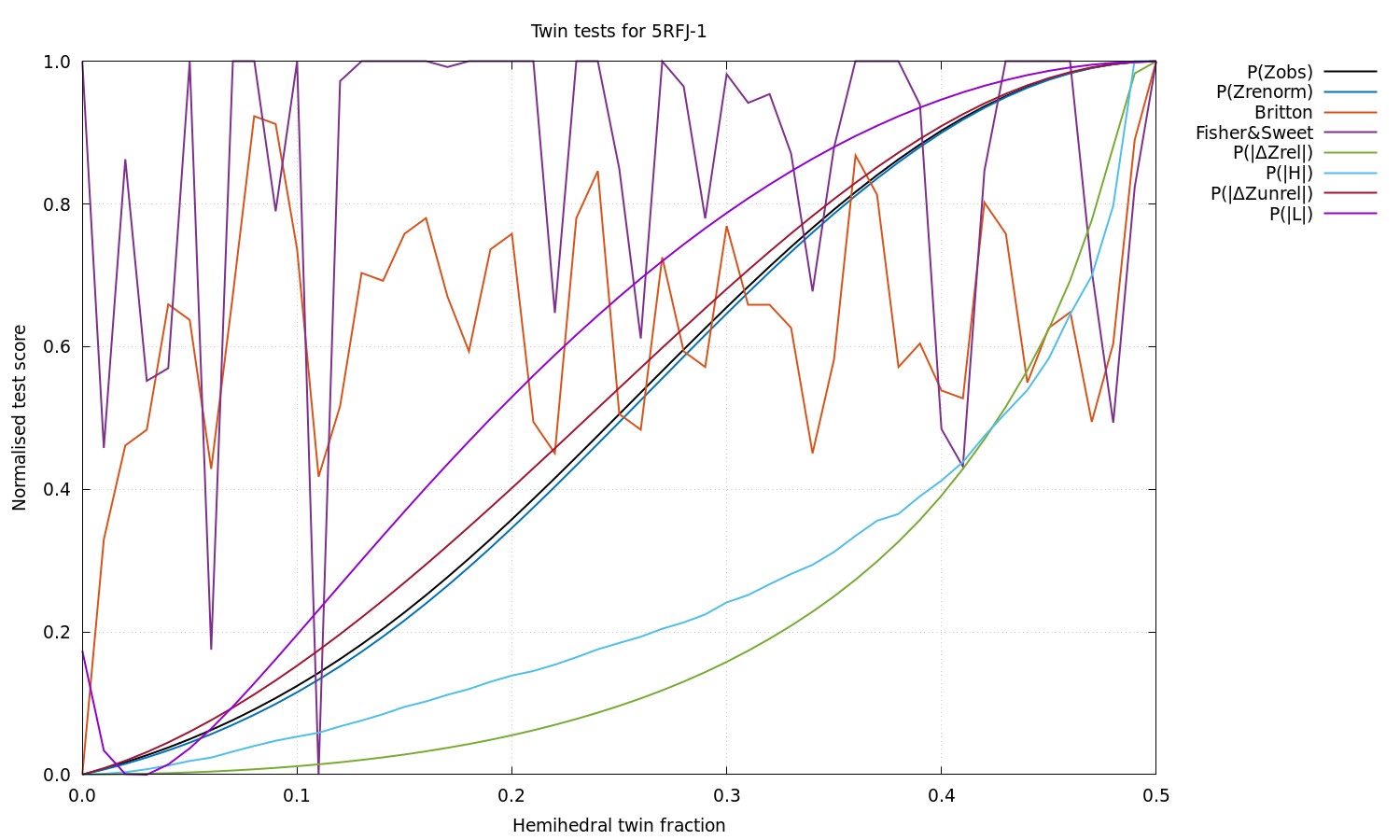### Anisotropy information extracted from the STARANISO log file for 5RFJ:

```Processing software:          XDS(integration)  Aimless(scaling)

Using MTZ column labels:      FP  SIGFP

NOTE: Not doing Bayesian estimation because corrected Is or Fs were input.

Unit cell and space group:      112.164   52.739   44.481  90.00 102.96  90.00   'C 1 2 1'
Nominal diffraction range:       54.654  1.800
Input reflection count:           23634
Unique reflection count:          23575

Diffraction cut-off criterion: Local mean I/sd(I) = 1.20

Diffraction limits & principal axes of ellipsoid fitted to diffraction cut-off surface:

2.025         0.9634   0.0000  -0.2679       0.981 a* - 0.193 c*
1.772         0.0000   1.0000   0.0000       b*
1.784         0.2679   0.0000   0.9634       0.609 a* + 0.793 c*

GoF to ellipsoid (d*):  0.0222    Fraction of surface points fitted:  100.0%  (     3870 /     3870)

Number of unobserved reflections inside ellipsoid:     1719
Number of observed reflections inside ellipsoid:      19573
Number of observed reflections outside ellipsoid:         5

Lowest cut-off diffraction limit:
2.135 at reflection  -44    0   15  in direction  -0.947 a* + 0.323 c*

Worst diffraction limit after cut-off:
2.192 at reflection  -44    0   14  in direction  -0.953 a* + 0.303 c*

Best diffraction limit after cut-off:
1.800 at reflection   -1   23   15  in direction  -0.036 a* + 0.837 b* + 0.546 c*

NOTE that because the cut-off surface is likely to be only very approximately ellipsoidal, in part
due to variations in reflection redundancy arising from the chosen collection strategy, the
directions of the worst and best diffraction limits may not correspond with the reciprocal axes,
even in high-symmetry space groups (the only constraint being that the surface must have point
symmetry at least that of the Laue class).

Fraction of data inside cut-off surface:                           83.0%  (    19578 /    23575)

Fraction of surface truncated by detector edges:                    2.4%  (       37 /     1572)

Fraction of total surface above threshold truncated by cusp(s):     0.0%  (        0 /     1574)

Scale:                       5.48456E-03    [ = factor to place Iobs on same scale as Iprofile/100.]
Beq:                               21.87    [ = equivalent overall isotropic B factor on Fs.]

B11      B22      B33      B23      B31      B12
Delta-B tensor:                               1.46    -0.80    -0.66     0.00     0.01     0.00

NOTE: The delta-B tensor is the overall anisotropy tensor on Fs after subtraction of Beq from its
diagonal elements (so trace = 0).

Eigenvalues (E) & eigenvectors of overall anisotropy (B) tensor on Fs:

23.33         1.0000   0.0000   0.0000       0.996 a* - 0.089 c*
21.07         0.0000   1.0000   0.0000       b*
21.20         0.0000   0.0000   1.0000       c*

The eigenvalues and eigenvectors of the overall B tensor are the squares of the lengths and the
directions of the principal axes of the ellipsoid that represents the tensor.

Delta-B eigenvalues:                     1.46    -0.80    -0.66

The delta-B eigenvalues are the eigenvalues of the overall anisotropy tensor after subtraction of
Beq (so sum = 0).

Angle & axis of rotation of diffraction-limit ellipsoid relative to anisotropy tensor:

15.54         0.0000   1.0000   0.0000

Anisotropy ratio:             0.103    [ = (Emax - Emin) / Beq ]
Fractional anisotropy:        0.058    [ = sqrt(1.5 Sum_i (E_i - Beq)^2 / Sum_i E_i^2) ]

Eigenvalues & eigenvectors of mean I/sd(I) anisotropy tensor:

1.04         0.9465   0.0000  -0.3228       0.976 a* - 0.216 c*
1.51         0.0000   1.0000   0.0000       b*
1.42         0.3228   0.0000   0.9465       0.692 a* + 0.722 c*

Eigenvalues & eigenvectors of weighted CC_1/2 anisotropy tensor:

0.188         0.9523   0.0000  -0.3053       0.978 a* - 0.208 c*
0.268         0.0000   1.0000   0.0000       b*
0.263         0.3053   0.0000   0.9523       0.667 a* + 0.745 c*

Eigenvalues & eigenvectors of mean K-L divergence anisotropy tensor:

0.358         0.9512   0.0000  -0.3086       0.978 a* - 0.210 c*
0.510         0.0000   1.0000   0.0000       b*
0.513         0.3086   0.0000   0.9512       0.672 a* + 0.741 c*

Ranges of local mean I/sd(I), local weighted CC_1/2, local mean K-L divergence and D-W factor [= exp(-4 pi^2 s~Us)]:

ISmean  CChalf  KLdive  DWfact
0   Grey                 Unobservable*
1   Blue                  Observable*
2   Red|Pink:9    1.20  0.3000   0.810  0.0887
3   Orange        3.02  0.8443   1.781  0.1811
4   Yellow        7.57  0.9639   2.956  0.3263
5   Green        15.25  0.9816   3.914  0.5195
6   Cyan         19.82  0.9847   4.293  0.7305
7   Magenta      25.97  0.9875   5.329  0.9076
8   White        29.22  0.9889   6.410  0.9961

* Refer to GLOSSARY for explanation of terminology.

The cut-off surface uses a different color scheme:
Unmeasured points are blue (inside the fitted surface) or cyan (outside).
Unobserved points are red (in) or green (out).
Observed points are orange (in) or white (out).
The fitted surface is magenta.

Anisotropic S/N ratio:         0.67    [ = max_h | exp(4 pi^2 s~_h delta(B) s_h) - 1 | <I_h/sd(I_h)> ]

The 'anisotropic S/N ratio', unlike the 'anisotropy ratio' or the 'fractional' anisotropy shown
above, in addition to the anisotropy of the B tensor, takes both the diffraction and the local mean
I/sd(I) into account.

Estimated twin fraction from K-L divergence of observed acentric Z probability (before, after):  0.00  0.00

Estimated twin fraction from Britton histogram for -h-l,-k,l operator (strong, all):             0.00  0.00

Estimated twin fraction from Murray-Rust plot for -h-l,-k,l operator (strong, all):              0.00  0.06

Estimated twin fraction from weighted Fisher & Sweet plot for -h-l,-k,l operator:                0.11

Estimated twin fraction from K-L divergence of |delta-Z| probability for -h-l,-k,l operator:     0.00

Estimated twin fraction from K-L divergence of |H| probability for -h-l,-k,l operator:           0.00

Estimated twin fraction from K-L divergence of unrelated acentric |delta-Z| probability:         0.00

Padilla & Yeates L test for twinning, acentric moments of |L|:
<|L|> (normal = .500; perfect twin = .375):  0.490
<L^2> (normal = .333; perfect twin = .200):  0.318

Estimated twin fraction from K-L divergence of |L| probability:                                  0.03

```

### Normalised scores from a panoply of hemihedral twinning tests.

For each test, the most likely hemihedral twin fraction based on the data is the one corresponding to the lowest normalised score (i.e. like the R value).  For all the distributional tests (i.e. those of the form P(x)), the raw score before normalisation is the Kullback-Leibler divergence from the theoretical to the observed probability density.  For the non-distributional Britton and Fisher & Sweet tests, it's the count of extra negative intensities obtained by detwinning the data using each possible twin fraction.  Note: click image to enlarge.Reference